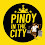## Pages

### Are we doing? - 하는 거야 / 거예요?

Are we doing? - 하는 거야 / 거예요?

하는 거야/ 거예요? is usually used when you want to ask someone what you (both singular and plural) are doing, or will be doing immediately.

For example, when you are in a group of people who are doing things as a group, and you want to ask a leader of the group what you all will be doing.

우리 저기 가는 거야? = Are we going there? [Informal]
우리 저기 가는 거예요? = Are we going there? [Polite]

Note: 하는 거야? = Are you/we doing?
HOWEVER, the polite form of "Are you doing?" usually uses "시는 거예요?" whereas the polite form of "Are we doing?" uses "는 거예요?"
See Are you doing? - ~는 거야? , Are you doing? - ~시는 거예요?

● Rule

Take 다 off a plain verb and add 는 거야 / 거예요?

• 하다 → 하는 거야 / 거예요? = Are we doing?
• 가다 → 가는 거야 / 거예요? = Are we going?
• 읽다 → 읽는 거야 / 거예요? = Are we reading?
• 보다 → 보는 거야 / 거예요? = Are we watching?
• 쓰다 → 쓰는 거야 / 거예요? = Are we writing?
• 먹다 → 먹는 거야 / 거예요? = Are we eating?
• 마시다 → 마시는 거야 / 거예요? = Are we drinking?
• 자다 → 자는 거야 / 거예요? = Are we sleeping?
• 보내다 → 보내는 거야 / 거예요? = Are we sending?
Example Sentences
• 운동 하는 거예요? = Are (we) exercising?
• 저기로 가는 거예요? = Are (we) going there?
• 이 차로 가는 거예요? = Are (we) going by this car?
• 이거 먹는 거예요? = Are (we) eating this?
• 이걸로 먹는 거예요? = Are (we) eating with this?
• 여기서 자는 거예요? = Are (we) sleeping here?
• 오늘 저 영화 보는 거예요? = Are (we) watching that movie today?
• 이걸로 보는 거예요? =  Are (we) watching with this?
You can add 언제, 어디, 누가, 뭐, 어떻게 or 왜 [when, where, who, what, how or why] in front of ~시는 거예요? to make these sentences.
• 오늘 우리 뭐 하는 거예요? = What are we doing today?
• 뭐 읽는 거예요? = What are (we) reading?
• 뭐 보는 거예요? = What are (we) watching?
• 뭐 쓰는 거예요? = What are (we) writing?
• 뭐 먹는 거예요? = What are (we) eating?
• 뭐 마시는 거예요? = What are (we) drinking?
• 뭐 보내는 거예요? = What are (we) sending?
• 어디 가는 거예요? = Where are (we) going?
• 어디서 자는 거예요? = Where are (we) sleeping?
• 누가 하는 거예요? = Who is doing?
• 어떻게 쓰는 거예요? = How are (we) writing?
• 왜 하는 거예요? = Why are (we) doing?
More Example Sentences
• 우리 어디 가는 거예요? = Where are we going?
• 아, 지금 시내에 가는 거예요. = Ah, we're going to the city.
• 이 차로 가는 거예요? = Are (we) going by this car?
• 네, 어서 타세요. = Yes, please get in.
• 뭐 먹을 거예요? = What will we eat?  Verbs - Future [Will]
• 스파게티 먹을 거예요. 스파게티 좋아하세요? = We will eat spaghetti. Do you like it?
• 네, 좋아해요. = Yes, I like it.

1.감사합니다.
이 사이트에 완전 좋은.

2.This is great. Thank you for your explanation.

I just have one question: What is the difference between -ㄹ 것이에요 and -는 것이에요?.

Both forms seem to be used for future...

I understand the differences among -겠- -ㄹ 게- and -ㄹ 것이다...

1.Hi Richardus,

-ㄹ 것이에요 means "I will do (something"

-는 것이에요? is a question which means "Is it doing (something)?"

For example,

나는 뉴욕에 갈 것이에요. = I will go to New York.

이 비행기는 뉴욕에 가는 것이에요? = Is this plane going to New York?

2.I'm slightly confused by use of -ㄹ 것이에요 for "I will do something)".

I know a couple ways of expressing the future, the two closest being -ㄹ 거예요 and -ㄹ 게요.

I thought 이에요 was used as "to be" (equality) and since 것 means "thing", the part 것이에요 means "is a thing" basically. So how does that tie in with -ㄹ to make future?

Somehow, I feel like I am missing something obvious. :)

3.Hi William,

-ㄹ 것이에요 is the written form, and -ㄹ 거예요 is the spoken form.

So, -ㄹ 거예요 is used more often when speaking.

Actually, -ㄹ 것이에요 is almost never used in spoken Korean.

Hope this helps.

Cheers.

3.Hi, first of all, I really like your blog. It is very useful. I have a question which I hope you can answer. What is the use of 해야 하는 거니? I came across it once, but I don't know how to translate it. Thanks a lot!